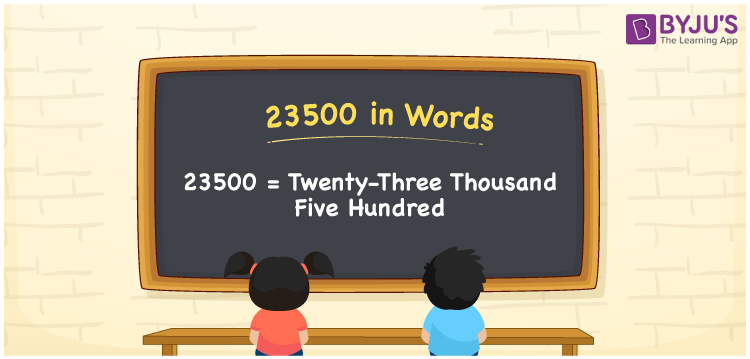# 23500 in Words

Twenty-three thousand five hundred is the number name for 23500. The place value chart is essential for converting numbers into words. As a result, we may conclude that the place value table is helpful when writing 23500 in words. For example, if you earned Rs. 23500 in 3 months, you may write, “I earned Rs. 23500 in three months”. Now let us take a closer look at how to write 23500 in words and the steps involved.

 23500 in Words: Twenty-three Thousand Five Hundred. Twenty-three Thousand Five Hundred in Numerical Form: 23500

## 23500 in English Words## How to Write 23500 in Words?

We can use a place value chart to convert 23500 to words. The place value table for the number 23500, as well as its expanded version, is shown below.

 Ten-thousands Thousands Hundreds Tens Ones 2 3 5 0 0

The number 23500 is written as follows using the place value chart:

= 2 × Ten thousand + 3 × Thousand + 5 × Hundred + 0 × Ten + 0 × One

= 2 × 10000 + 3 × 1000 + 5 × 100

= 20000 + 3000 + 500

= 23500

= twenty-three thousand five hundred

Hence, 23500 in words is twenty-three thousand five hundred.

After 23499 but before 23501, the natural number 23500 appears.

23500 in words – Twenty-three thousand five hundred

Is 23500 an odd number? – No

Is 23500 an even number? – Yes

Is 23500 a perfect square number? – No

Is 23500 a perfect cube number? – No

Is 23500 a prime number? – No

Is 23500 a composite number? – Yes

## Frequently Asked Questions on 23500 in Words

Q1

### How to write 23500 in words?

23500 in words is twenty-three thousand five hundred.

Q2

### Simplify 20000 + 3500, and express in words.

Simplifying 20000 + 3500, we get 23500. Hence, 23500 in words is twenty-three thousand five hundred.

Q3

### Write twenty-three thousand five hundred in numbers.

Twenty-three thousand five hundred in numbers is 23500.